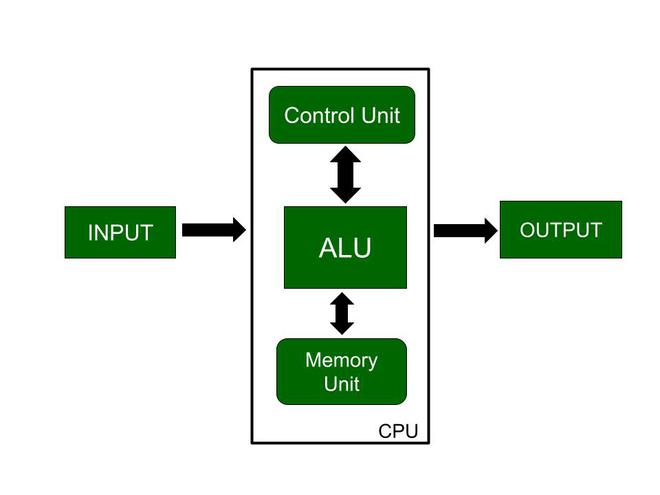Related Articles

# Central Processing Unit (CPU)

• Last Updated : 16 Jun, 2021

CPU is the brain of the computer. All types of data processing operations and all the important functions of a computer are performed by the CPU. It helps input and output devices to communicate with each other and perform their respective operations. It also stores data which is input, intermediate results in between processing, and instructions.

Now, the CPU consists of 3 major units, which are:

1. Memory or Storage Unit
2. Control Unit
3. ALU(Arithmetic Logic Unit)

Let us now look at the block diagram of the computer:Here, in this diagram, the three major components are also shown. So, let us discuss these major components:

Memory or Storage Unit

As the name suggests this unit can store instructions, data, and intermediate results. The memory unit is responsible for transferring information to other units of the computer when needed. It is also known as an internal storage unit or the main memory or the primary storage or Random Access Memory (RAM) as all these are storage devices.

Its size affects speed, power, and performance. There are two types of memory in the computer, which are primary memory and secondary memory. Some main functions of memory units are listed below:

• Data and instructions are stored in memory units which are required for processing.
• It also stores the intermediate results of any calculation or task when they are in process.
• The final results of processing are stored in the memory units before these results are released to an output device for giving the output to the user.
• All sorts of inputs and outputs are transmitted through the memory unit.

Control Unit

As the name suggests, a control unit controls the operations of all parts of the computer but it does not carry out any data processing operations. For executing already stored instructions, It instructs the computer by using the electrical signals to instruct the computer system. It takes instructions from the memory unit and then decodes the instructions after that it executes those instructions. So, it controls the functioning of the computer. It’s main task is to maintain the flow of information across the processor. Some main functions of the control unit are listed below:

• Controlling of data and transfer of data and instructions is done by the control unit among other parts of the computer.
• The control unit is responsible for managing all the units of the computer.
• The main task of the control unit is to obtain the instructions or data which is input from the memory unit, interprets them, and then directs the operation of the computer according to that.
• The control unit is responsible for communication with Input and output devices for the transfer of data or results from memory.
• The control unit is not responsible for the processing of data or storing data.

ALU (Arithmetic Logic Unit)

ALU (Arithmetic Logic Unit) is responsible for performing arithmetic and logical functions or operations. It consists of two subsections, which are:

• Arithmetic Section
• Logic Section

Now, let us know about these subsections:

Arithmetic Section: By arithmetic operations, we mean operations like addition, subtraction, multiplication, and division, and all these operation and functions are performed by ALU. Also, all the complex operations are done by making repetitive use of the mentioned operations by ALU.

Logic Section: By Logical operations, we mean operations or functions like selecting, comparing, matching, and merging the data, and all these are performed by ALU.

Note: CPU may contain more than one ALU and ALUs can be used for maintaining timers that help run the computer system.

### Sample Questions

Question 1. Which of the following are the major components of the CPU?

(A) Memory or Storage Unit

(B) Control Unit

(C) ALU(Arithmetic Logic Unit)

(D) All of the above

Solution:

The correct option is A, i.e., All of the above

The CPU consists of 3 major units which are: Memory or Storage Unit, Control Unit, ALU(Arithmetic Logic Unit).

Question 2. All sorts of inputs and outputs are transmitted through the ___________.

(A) Memory unit

(B) ALU

(C) Control unit

(D) None of the above

Solution:

The correct option is A, i.e, Memory unit.

All sorts of inputs and outputs are transmitted through the memory unit.

Question 3.  __________ is not responsible for processing of data or to store data.

(A) Memory unit

(B) ALU

(C) Control unit

(D) None of the above

Solution:

The correct option is C, i.e., Control unit

The control unit is not responsible for processing data or storing data.

Question 4. Comparing operation is which type of operation?

(A) Arithmetic

(B) Logical

(C) both a and b

(d) None of the above

Solution:

The correct option is B, i.e., Logical

By logical operations, we mean operations or functions like selecting, comparing, matching, and merging the data and all these are performed by ALU.

Question 5. ______________  is responsible for communication with Input and output devices for transfer of data or results from memory.

(A) Memory unit

(B) ALU

(C) Control unit

(D) None of the above

Solution:

The correct option is C, i.e, Control unit

The control unit is responsible for communication with input and output devices for the transfer of data or results from memory.

Attention reader! Don’t stop learning now. Join the First-Step-to-DSA Course for Class 9 to 12 students , specifically designed to introduce data structures and algorithms to the class 9 to 12 students

My Personal Notes arrow_drop_up